# VBA Solver

Last updated on November 23, 2021

This tutorial will show you how to use the Solver add-in in VBA.

Solver is an add-in that is provided with Excel and is used to perform ‘what-if’ analysis by providing alternative answers to a formula in a cell based on values that you may pass to the formula from other cells in your workbook.

## Enabling the Solver Add-In in Excel

Select the File on the Excel ribbon and then go down to Options.Select Add-ins and click on the Go button next to Excel Add-ins.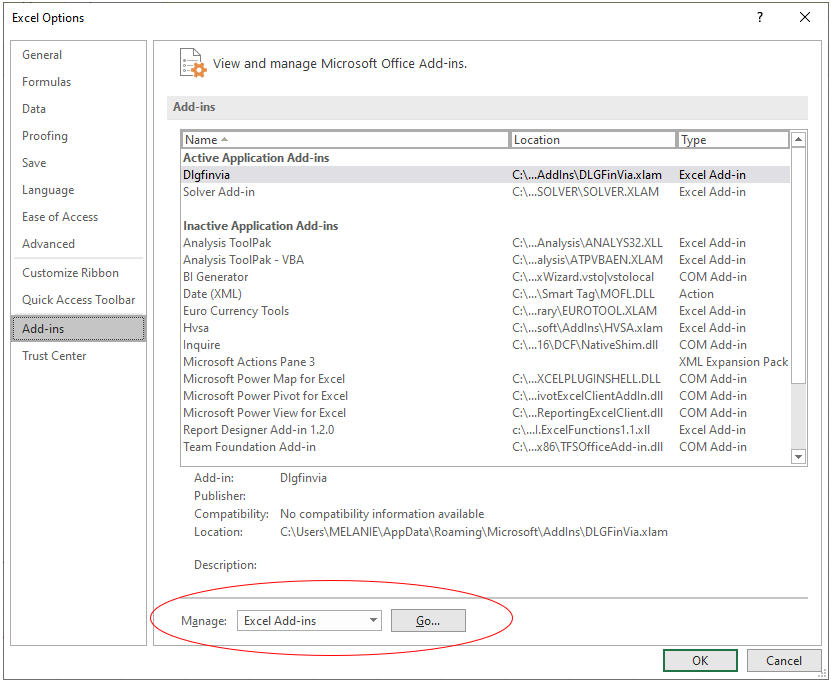Make sure the Solver Add-in option is selected.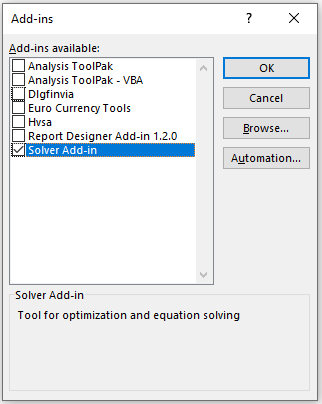Alternatively, click on the Excel Add-ins on the Developer ribbon to get the Add-ins dialog box.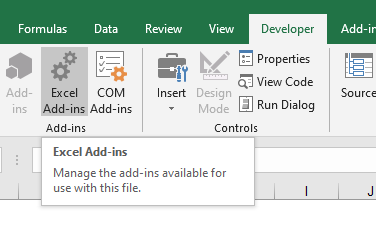## Enabling the Solver Add-in in VBA

Once you have enabled the Solver Add-in in Excel, you then need to add a reference to it in your VBA Project in order to use it in VBA.

Make sure you are clicked in the VBA Project where you wish to use the Solver. Click on the Tools menu and then on References.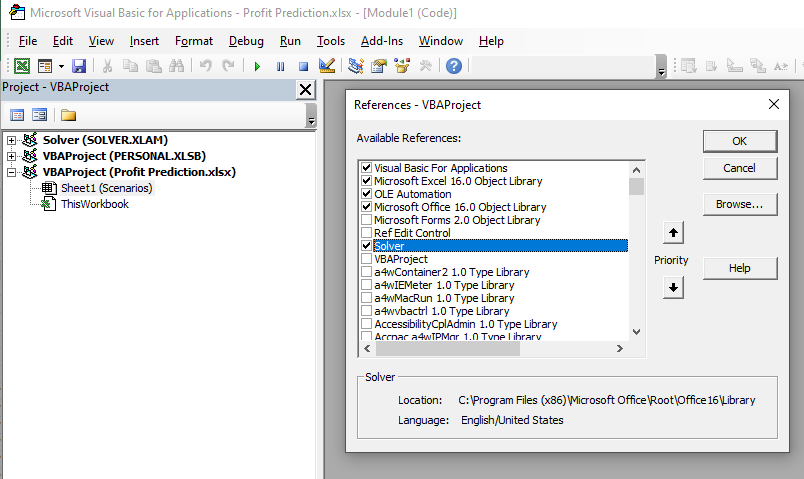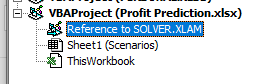You can now use the Solver Add-in in VBA code!

## Using Solver Functions in VBA

We need to use 3 Solver VBA functions to use Solver in VBA. These are  SolverOK, SolverAdd, and SolverSolve.

### SolverOK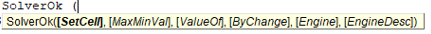• SetCelloptional – this needs to refer to the cell that needs to be changed – it needs to contain a formula. This corresponds to the Set Objective Cell box in the Solver Parameters dialog box.
• MaxMinVal optional – You can set this to 1 (Maximize), 2 (Minimize) or 3. This  corresponds to the MaxMin, and Value options in the Solver Parameters dialog box.
• ValueOf  – optional -If the MaxMinValue is set to 3, then you need to supply this argument.
• ByChange – optional -This tells solver which cells it may change in order to get to the required value. This corresponds to the By Changing Variable Cells box in the Solver Parameters dialog box.
• Engineoptional –  this indicates the solving method that needs to be used to get to a solution.  1 for the Simplex LP method, 2 for the GRG Nonlinear method, or 3 for the Evolutionary method.  This corresponds to the Select a Solving Method dropdown list in the Solver Parameters dialog box
• EngineDesc optional -this is an alternate way of selecting the solving method  – here you would type the strings “Simplex LP”, “GRG Nonlinear” or “Evolutionary”.  This also corresponds to the Select a Solving Method dropdown list in the Solver Parameters dialog box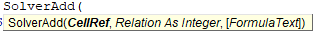• CellRefrequiredthis is a reference to a cell or a range of cells that are to be changed to solve the problem.
• Relationrequired – this is an integer which has to be between 1 to 6 and specifies the logical relation allowed.
• 1 is less than (<=)
• 2 is equal to (=)
• 3 is greater than (>=)
• 4 is must have final values that are integers.
• 5 is must have values between 0 or 1.
• 6 is must have final values that are all different and integers.
• FormulaText optional – The right side of the constraint.

## Creating a Solver Example

Consider the following worksheet.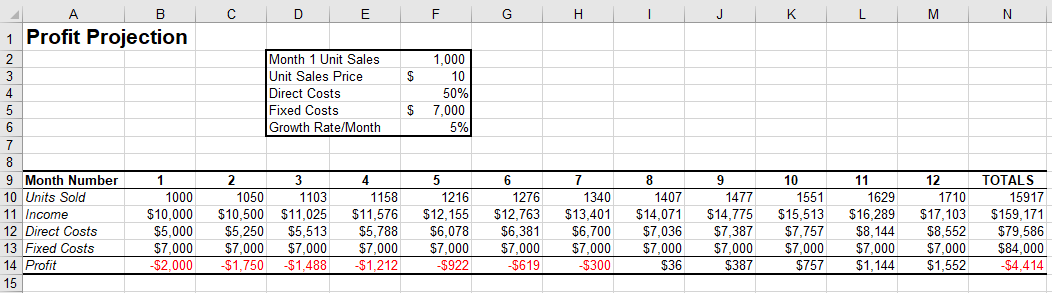In the sheet above, we need to break even in Month Number one by setting cell B14 to zero by amending the criteria in cells F1 to F6.

``````Sub TestSolver
SolverOk SetCell:="\$B\$14", MaxMinVal:=3, ValueOf:=0, ByChange:="\$F\$2:\$F\$6", Engine:=1, EngineDesc:="GRG Nonlinear"
End Sub``````

Once you have set up the SolverOK parameters, you need to add some criteria restrictions.

``````Sub TestSolver
SolverOk SetCell:="\$B\$14", MaxMinVal:=3, ValueOf:=0, ByChange:="\$F\$2:\$F\$6", Engine:=1, EngineDesc:="GRG Nonlinear"
'add criteria - F3 cannot be less than 8
'add criteria - F5 cannot be less than 5000
End Sub``````

Once you have set the SolverOK and the SolverAdd (if required), you can Solve the problem.

``````Sub TestSolver
SolverOk SetCell:="\$B\$14", MaxMinVal:=3, ValueOf:=0, ByChange:="\$F\$2:\$F\$6", Engine:=1, EngineDesc:="GRG Nonlinear"
'add criteria - F3 cannot be less than 8 SolverAdd CellRef:="\$F\$3", Relation:=3, FormulaText:="8" 'add criteria - F3 cannot be less than 5000
'find a solution by solving the problem
SolverSolve
End Sub``````

Once you run the code, the following window will show on your screen.   Select the option you require (ie Keep the Solver solution, or Restore Original Values), and click OK.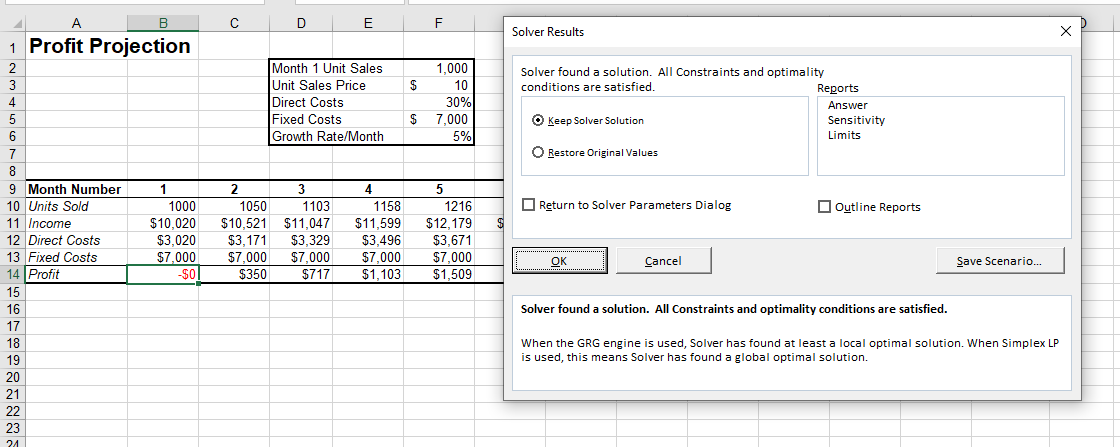Stop searching for VBA code online. Learn more about AutoMacro - A VBA Code Builder that allows beginners to code procedures from scratch with minimal coding knowledge and with many time-saving features for all users!